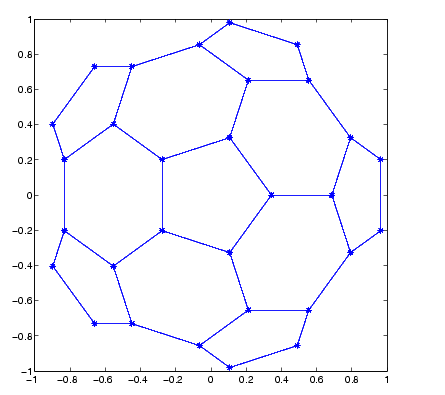MATLAB Function Referencegplot

Plot set of nodes using an adjacency matrix

Syntax

• ```gplot(A,Coordinates)
gplot(A,Coordinates,`LineSpec`)
```

Description

The `gplot` function graphs a set of coordinates using an adjacency matrix.

```gplot(A,Coordinates) ``` plots a graph of the nodes defined in `Coordinates` according to the n-by-n adjacency matrix `A`, where n is the number of nodes. `Coordinates` is an n-by-2 matrix, where n is the number of nodes and each coordinate pair represents one node.

```gplot(A,Coordinates,LineSpec) ``` plots the nodes using the line type, marker symbol, and color specified by `LineSpec`.

Remarks

For two-dimensional data, `Coordinates(i,:)` `=` `[x(i)` `y(i)]` denotes node `i`, and `Coordinates(j,:)` `=` `[x(j)` `y(j)]` denotes node `j`. If node `i` and node `j` are connected, `A(i`,`j)` or `A(j`,`i)` is nonzero; otherwise, `A(i`,`j)` and `A(j`,`i)` are zero.

Examples

To draw half of a Bucky ball with asterisks at each node,

• ```k = 1:30;
[B,XY] = bucky;
gplot(B(k,k),XY(k,:),'-*')
axis square```

See Also

`LineSpec`, `sparse`, `spy`

Tree Operations for related functions

© 1994-2005 The MathWorks, Inc.